# Tag Archives: Monotone EQ Sat

## Monotone EQSat

Today I’m posting 2 problems relating to my student Dan Thornton’s independent study work.  I’m out of town this week and next, so I have these posts set to automatically go up on Tuesday afternoon.

Dan’s independent study was based on the “Automaton Identification” problem, but to show that reduction, he needs to use a variant of 3SAT, which he shows here:

The problem: Monotone EQ SAT. This is a specific instance of Monotone SAT.

The description:
We are given a conjunction of clauses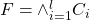where each clause in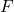contains all negated or non-negated variables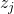and the number of clauses and variables are equal, is there an assignment of the variables so thatis satisfied? Our instance will haveclauses and variables.

Example:
Here is anthat has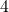variables andclauses.

F =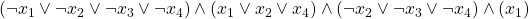The abovemay be satisfied by the following assignment: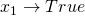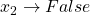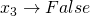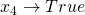The reduction:
We will reduce from Monotone SAT. So we are given an instance of Monotone  SAT with the clauses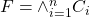here each clause is of the form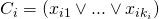where each clause has all negated or non-negated variables. This is different from Monotone EQ SAT as we do not require the number of variables and clauses to be equal.
From thiswe must build an instance of Monotone EQ SAT.
We may transform our instance of Monotone SAT,, into one of Monotone EQ SAT by the following iterative procedure. New variables will be denoted by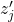and new clauses by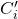.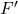=;
i = 1;
j = 1;
While{number of clauses != number of variables}{
introduce two new variables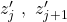;
If{number of variables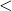number of clauses}{
Create the new clause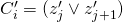;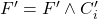;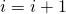;
}
else {
Create three new clauses: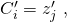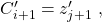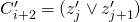;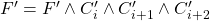;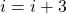;
}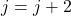;
}



The above algorithm will produce an equationthat is in Monotone EQ  SAT. This may be shown by induction. Notice that before the procedure if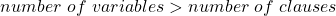that we will add 2 new variables and 3 new clauses.

If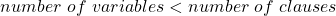then we will add 2 new variables but only a single new clause. Either way the difference between the number of variables and clauses,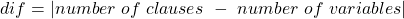will decrease by. So in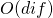steps we will obtain an formula where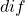= 0. Such an formula is an instance of Monotone EQ SAT.

True{Monotone SAT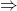True{Monotone EQ SAT}
Here we assume that there is a truth assignment function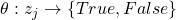that maps every variable to a truth value, such thatis satisfied. Then after we preform the above algorithm we have an instance of, now our instance ofwill be of the form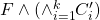for some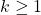. Now notice that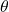above will satisfyinand we may trivially satisfy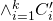by simply assigning all new variablesto true.
This will give us a new truth assignment function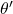that will satisfyTrue{Monotone EQ SAT}True{Monotone SAT}
Here we assume that there is a truth assignment function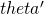that will satisfythen obviously as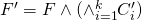thenmust also satisfy.

(Back to me)

Difficulty: 3.  The logical manipulations aren’t hard, but it is possible to mess them up.  For example, it’s important that the algorithm above reduces the difference in variables and clauses by 1 each iteration.  If it can reduce by more, you run the risk of skipping over the EQ state.